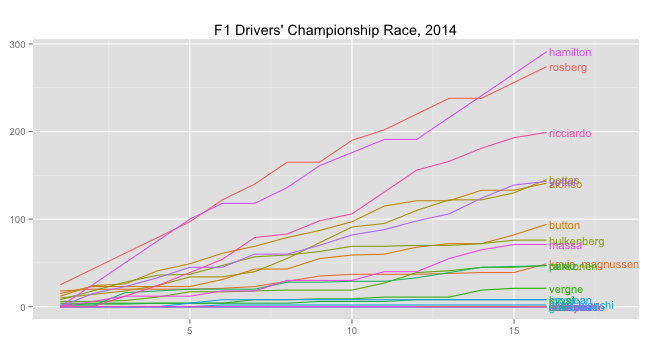## Sunday, November 2, 2014

### The 2014 Drivers' Championship Race Going in to Round 17

Some quick doodles for a new chapter of Wrangling F1 Data With R, looking at the state of the drivers' championship race as we go in to round 17.

```#Load in the core utility functions to access ergast API
source('ergastR-core.R')

#Get the standings after each of the first 16 rounds of the 2014 season
df=data.frame()
for (j in seq(1,16)){
dft=seasonStandings(2014,j)
dft\$round=j
df=rbind(df,dft)
}
#Data is now in: df
```

Now we can have a look at the data. First, the race in the style of a lapchart, plotting the position standings after each round.

```library(ggplot2)
library(directlabels)

#The base chart
g=ggplot(df,aes(x=round,y=pos,col=driverId))
g=g+geom_line()
#Remove axis labels and colour legend
g=g+ylab(NULL)+xlab(NULL)+guides(color=FALSE)
g=g+ggtitle("F1 Drivers' Championship Race, 2014")
#Add the line labels, resized (cex), and with an x-value offset
g=g+geom_dl(aes(label=driverId),list("last.points",cex=0.7,dl.trans(x=x+0.1)))
#Add right hand side padding to the chart so the labels don't overflow
g=g+scale_x_continuous(limits=c(1,18))
```

This chart shows competition throughout the season  particularly between the first two places (Rosberg and Hamilton), fourth to sixth (Bottas, Vettel and Alonso), and ten, eleventh and twelfth (Magnussen, Perez and Raikkonen).

We can get a better feel for the competition in terms of the number of points separating the drivers.

```#The only difference if to the base chart
g=ggplot(df,aes(x=round,y=points,col=driverId))
#All the other elements of the chart definition are the same```(Note there is some occlusion of the labels which we would need to manage by hand using the directlabels dl.move() function applying the necessary vjust offset to each driverId group (e.g. alonso).)

Here we see how close fought the fourth to sixth battle has become, as the the points battle for tenth place. We also see a late season charge from Massa, who could still challenge Hulkenberg for eighth.

Let's annotate the chart a little more by placing a guideline showing between 10th and 11th positions.

`#Generate a guide that is the mean points value of 10th and 11th positions`
`dfx=ddply(df[df['pos']==10 | df['pos']==11,],`
`  .(round), summarize, points=mean(points))`
`dfx\$driverId=''`

`#Get the drivers fighting around 10th at the end of round 16`
```#Note that other drivers may have contended this position earlier in the season
df.battle=df[df\$driverId %in% as.character(df[df\$round==16 & df\$pos>=10 & df\$pos<=12,'driverId']),]
#Base chart
g=ggplot(df.battle,aes(x=round,y=points,col=driverId))
g=g+geom_line()
g=g+ylab(NULL)+xlab(NULL)+ggtitle("F1 Drivers' Championship Race, 2014")+guides(color=FALSE)
g=g+geom_dl(aes(label=driverId),list("last.points",cex=0.7,dl.trans(x=x+0.1)))
g=g+scale_x_continuous(limits=c(1,18))